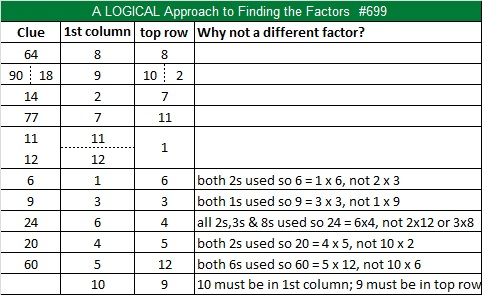# Level 4 Christmas Puzzle #699

• 699 is a composite number.
• Prime factorization: 699 = 3 x 233
• The exponents in the prime factorization are 1 and 1. Adding one to each and multiplying we get (1 + 1)(1 + 1) = 2 x 2 = 4. Therefore 699 has exactly 4 factors.
• Factors of 699: 1, 3, 233, 699
• Factor pairs: 699 = 1 x 699 or 3 x 233
• 699 has no square factors that allow its square root to be simplified. √699 ≈ 26.438608.Here is a Christmas puzzle for you to solve. It’s numbered 699 to distinguish it from every other puzzle I make:Print the puzzles or type the solution on this excel file: 12 Factors 2015-11-30

———————————————————————————

699 is the smallest number whose digits add up to 24.

Every odd number greater than 1 is the sum of 2 consecutive numbers. 699 is the sum of 349 and 350.

Every number that is divisible by 3 is the sum of 3 consecutive numbers: 232 + 233 + 234 = 699.

Also 699 is the hypotenuse of Pythagorean triple 315-624-699. Which factor of 699 is the greatest common factor of those three numbers?

699 is palindrome 272 in BASE 17; note that 2(289) + 7(17) + 2(1) = 699

———————————————————————————This site uses Akismet to reduce spam. Learn how your comment data is processed.